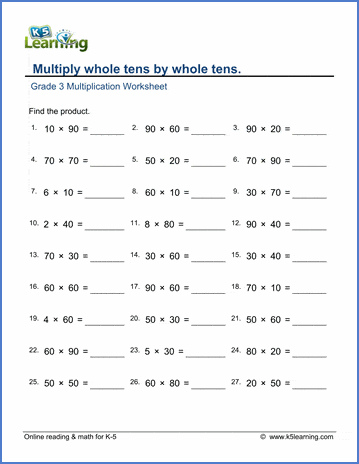# Multiply whole tens by whole tens

## Math worksheets: Multiplying whole tens (10-90) by whole tens (10-90)

Below are six versions of our grade 3 multiplication worksheet on multiplying whole tens by whole tens.  Equations with single digit numbers multiplying whole tens are also included.  These worksheets are pdf files.## More multiplication worksheets

Find all of our multiplication worksheets, from basic multiplication facts to multiplying multi-digit whole numbers in columns.

## What is K5?

K5 Learning offers reading and math worksheets, workbooks and an online reading and math program for kids in kindergarten to grade 5.  We help your children build good study habits and excel in school.